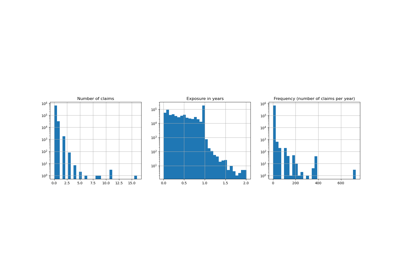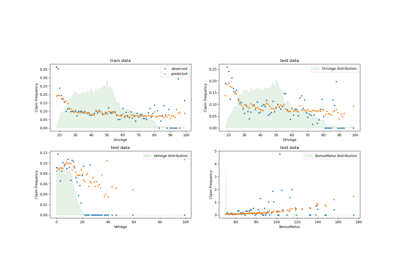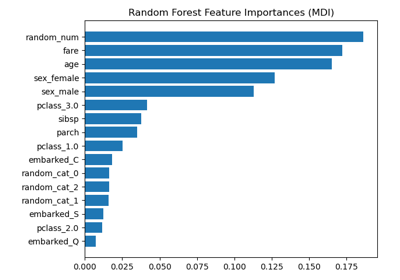# sklearn.compose.ColumnTransformer¶

class sklearn.compose.ColumnTransformer(transformers, *, remainder='drop', sparse_threshold=0.3, n_jobs=None, transformer_weights=None, verbose=False)[source]

Applies transformers to columns of an array or pandas DataFrame.

This estimator allows different columns or column subsets of the input to be transformed separately and the features generated by each transformer will be concatenated to form a single feature space. This is useful for heterogeneous or columnar data, to combine several feature extraction mechanisms or transformations into a single transformer.

Read more in the User Guide.

New in version 0.20.

Parameters
transformerslist of tuples

List of (name, transformer, columns) tuples specifying the transformer objects to be applied to subsets of the data.

namestr

Like in Pipeline and FeatureUnion, this allows the transformer and its parameters to be set using set_params and searched in grid search.

transformer{‘drop’, ‘passthrough’} or estimator

Estimator must support fit and transform. Special-cased strings ‘drop’ and ‘passthrough’ are accepted as well, to indicate to drop the columns or to pass them through untransformed, respectively.

columnsstr, array-like of str, int, array-like of int, array-like of bool, slice or callable

Indexes the data on its second axis. Integers are interpreted as positional columns, while strings can reference DataFrame columns by name. A scalar string or int should be used where transformer expects X to be a 1d array-like (vector), otherwise a 2d array will be passed to the transformer. A callable is passed the input data X and can return any of the above. To select multiple columns by name or dtype, you can use make_column_selector.

remainder{‘drop’, ‘passthrough’} or estimator, default=’drop’

By default, only the specified columns in transformers are transformed and combined in the output, and the non-specified columns are dropped. (default of 'drop'). By specifying remainder='passthrough', all remaining columns that were not specified in transformers will be automatically passed through. This subset of columns is concatenated with the output of the transformers. By setting remainder to be an estimator, the remaining non-specified columns will use the remainder estimator. The estimator must support fit and transform. Note that using this feature requires that the DataFrame columns input at fit and transform have identical order.

sparse_thresholdfloat, default=0.3

If the output of the different transformers contains sparse matrices, these will be stacked as a sparse matrix if the overall density is lower than this value. Use sparse_threshold=0 to always return dense. When the transformed output consists of all dense data, the stacked result will be dense, and this keyword will be ignored.

n_jobsint, default=None

Number of jobs to run in parallel. None means 1 unless in a joblib.parallel_backend context. -1 means using all processors. See Glossary for more details.

transformer_weightsdict, default=None

Multiplicative weights for features per transformer. The output of the transformer is multiplied by these weights. Keys are transformer names, values the weights.

verbosebool, default=False

If True, the time elapsed while fitting each transformer will be printed as it is completed.

Attributes
transformers_list

The collection of fitted transformers as tuples of (name, fitted_transformer, column). fitted_transformer can be an estimator, ‘drop’, or ‘passthrough’. In case there were no columns selected, this will be the unfitted transformer. If there are remaining columns, the final element is a tuple of the form: (‘remainder’, transformer, remaining_columns) corresponding to the remainder parameter. If there are remaining columns, then len(transformers_)==len(transformers)+1, otherwise len(transformers_)==len(transformers).

named_transformers_Bunch

Access the fitted transformer by name.

sparse_output_bool

Boolean flag indicating whether the output of transform is a sparse matrix or a dense numpy array, which depends on the output of the individual transformers and the sparse_threshold keyword.

sklearn.compose.make_column_transformer

convenience function for combining the outputs of multiple transformer objects applied to column subsets of the original feature space.

sklearn.compose.make_column_selector

convenience function for selecting columns based on datatype or the columns name with a regex pattern.

Notes

The order of the columns in the transformed feature matrix follows the order of how the columns are specified in the transformers list. Columns of the original feature matrix that are not specified are dropped from the resulting transformed feature matrix, unless specified in the passthrough keyword. Those columns specified with passthrough are added at the right to the output of the transformers.

Examples

>>> import numpy as np
>>> from sklearn.compose import ColumnTransformer
>>> from sklearn.preprocessing import Normalizer
>>> ct = ColumnTransformer(
...     [("norm1", Normalizer(norm='l1'), [0, 1]),
...      ("norm2", Normalizer(norm='l1'), slice(2, 4))])
>>> X = np.array([[0., 1., 2., 2.],
...               [1., 1., 0., 1.]])
>>> # Normalizer scales each row of X to unit norm. A separate scaling
>>> # is applied for the two first and two last elements of each
>>> # row independently.
>>> ct.fit_transform(X)
array([[0. , 1. , 0.5, 0.5],
[0.5, 0.5, 0. , 1. ]])


Methods

 fit(X[, y]) Fit all transformers using X. fit_transform(X[, y]) Fit all transformers, transform the data and concatenate results. Get feature names from all transformers. get_params([deep]) Get parameters for this estimator. set_params(**kwargs) Set the parameters of this estimator. Transform X separately by each transformer, concatenate results.
__init__(transformers, *, remainder='drop', sparse_threshold=0.3, n_jobs=None, transformer_weights=None, verbose=False)[source]

Initialize self. See help(type(self)) for accurate signature.

fit(X, y=None)[source]

Fit all transformers using X.

Parameters
X{array-like, dataframe} of shape (n_samples, n_features)

Input data, of which specified subsets are used to fit the transformers.

yarray-like of shape (n_samples,…), default=None

Targets for supervised learning.

Returns
selfColumnTransformer

This estimator

fit_transform(X, y=None)[source]

Fit all transformers, transform the data and concatenate results.

Parameters
X{array-like, dataframe} of shape (n_samples, n_features)

Input data, of which specified subsets are used to fit the transformers.

yarray-like of shape (n_samples,), default=None

Targets for supervised learning.

Returns
X_t{array-like, sparse matrix} of shape (n_samples, sum_n_components)

hstack of results of transformers. sum_n_components is the sum of n_components (output dimension) over transformers. If any result is a sparse matrix, everything will be converted to sparse matrices.

get_feature_names()[source]

Get feature names from all transformers.

Returns
feature_nameslist of strings

Names of the features produced by transform.

get_params(deep=True)[source]

Get parameters for this estimator.

Parameters
deepbool, default=True

If True, will return the parameters for this estimator and contained subobjects that are estimators.

Returns
paramsdict

Parameter names mapped to their values.

property named_transformers_

Access the fitted transformer by name.

Read-only attribute to access any transformer by given name. Keys are transformer names and values are the fitted transformer objects.

set_params(**kwargs)[source]

Set the parameters of this estimator.

Valid parameter keys can be listed with get_params().

Returns
self
transform(X)[source]

Transform X separately by each transformer, concatenate results.

Parameters
X{array-like, dataframe} of shape (n_samples, n_features)

The data to be transformed by subset.

Returns
X_t{array-like, sparse matrix} of shape (n_samples, sum_n_components)

hstack of results of transformers. sum_n_components is the sum of n_components (output dimension) over transformers. If any result is a sparse matrix, everything will be converted to sparse matrices.

## Examples using sklearn.compose.ColumnTransformer¶